# 1.用 JavaScript 实现链表## 节点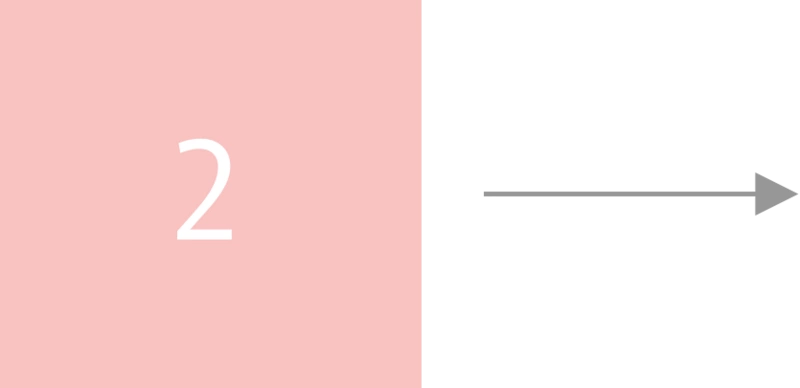• 指向链表中下一项的指针或引用(对于单链表)

• 节点的值

## 节点链表• push(value): 将值添加到链表的末尾

• pop() ：弹出链表中的最后一个值

• get(index)：返回给定索引中的项

• delete(index)：从给定索引中删除项

• isEmpty(): 返回一个布尔值，指示链表是否为空

printList():不是链表的原生方法，它将打印出我们的链表，主要用于调试

## 构造函数

• tail:对链表末尾节点的引用

• length:链表中有多少节点

constructor() {
this.tail = null;
this.length = 0;
}
}

## IsEmpty

`isEmpty()` 方法是一个帮助函数，如果链表为空，则返回`true`

```isEmpty() {
return this.length === 0;
}```

## printList

```printList () {
const nodes = [];
while (current) {
nodes.push(current.value);
current = current.next;
}
return nodes.join(' -> ');
}```

## Push

• `isEmpty()`方法返回`true`(链表的长度为零)

• `head` 指针为空

```if (this.head === null) {
this.tail = node;
this.length++;
return node;
}```

• `tail.next` 指向新节点

• 将 `tail` 指向新节点

• 更新链表长度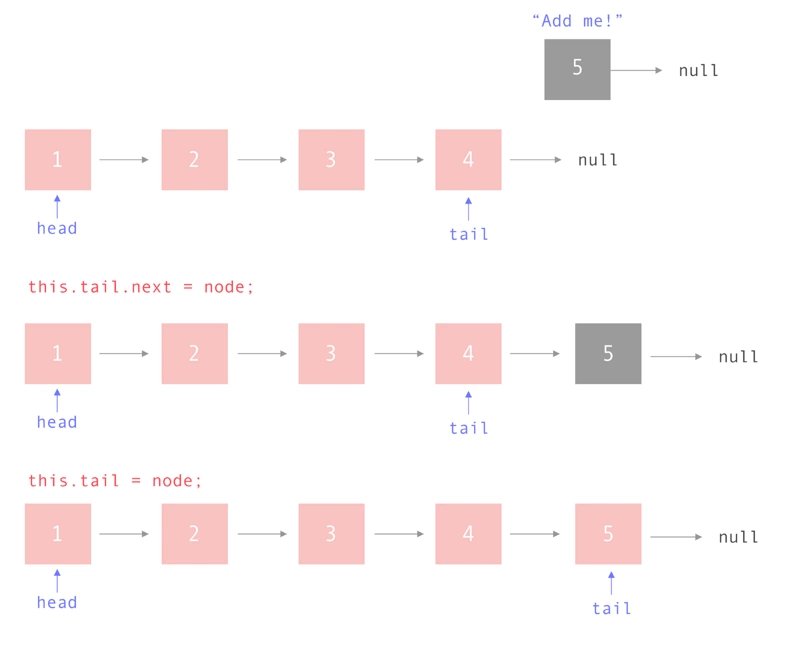```push(value) {
const node = Node(value);
// The list is empty
this.tail = node;
this.length++;
return node;
}
this.tail.next = node;
this.tail = node;
this.length++;
}```

## Pop

• 检查链表是否为空

• 检查链表中是否只有一项

```if (this.isEmpty()) {
return null;
}```

```if (this.head === this.tail) {
this.tail = null;
this.length--;
return nodeToRemove;
}```

```当链表中有节点时，
如果链表中的下一个节点是 tail
更新 tail 指向当前节点
当前节点设置为 null，
更新链表的长度
返回前一个 tail 元素```

```    1  let currentNode = this.head;
2  let secondToLastNode;
3
4  //从前面开始并迭代直到找到倒数第二个节点
5
6  while (currentNode) {
7    if (currentNode.next === this.tail) {
8      // 将第二个节点的指针移动到最后一个节点
9      secondToLastNode = currentNode;
10      break;
11    }
12    currentNode = currentNode.next;
13  }
14  // 弹出该节点
15  secondToLastNode.next = null;
16  // 将 tail 移动到倒数第二个节点
17  this.tail = secondToLastNode;
18  this.length--;
19
20  // 初始化 this.tail
21   return nodeToRemove;```

```if (currentNode.next === this.tail) {
secondToLastNode = currentNode;
}```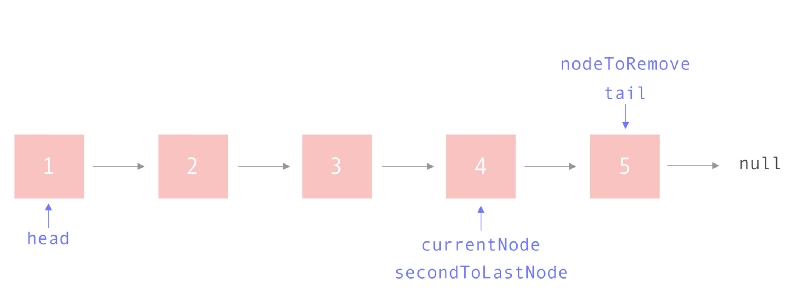`secondToLastNode.next = null;`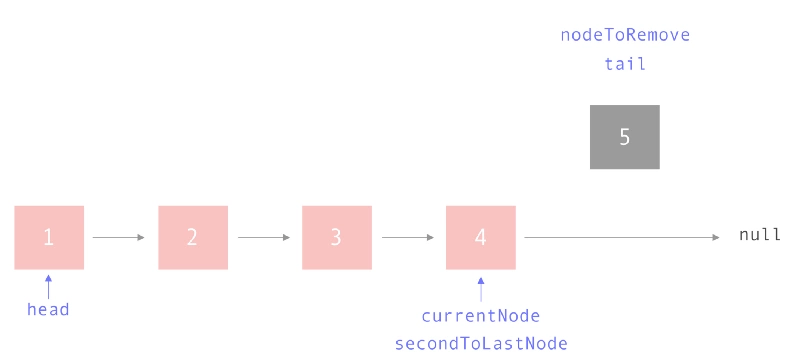`this.tail = secondToLastNode;`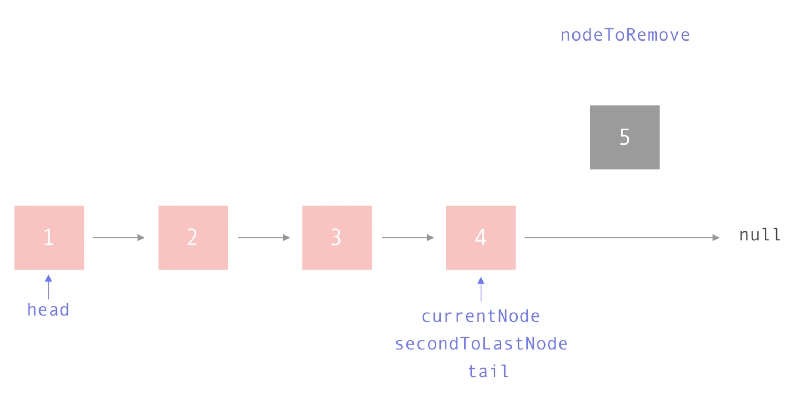```pop() {
if (this.isEmpty()) {
return null;
}
const nodeToRemove = this.tail;
// There's only one node!
this.tail = null;
this.length--;
return nodeToRemove;
}

let secondToLastNode;

// Start at the front and iterate until
// we find the second to last node
while (currentNode) {
if (currentNode.next === this.tail) {
// Move the pointer for the second to last node
secondToLastNode = currentNode;
break;
}
currentNode = currentNode.next;
}
// Pop off that node
secondToLastNode.next = null;
// Move the tail to the second to last node
this.tail = secondToLastNode;
this.length--;

// Initialized to this.tail
return nodeToRemove;
}```

## Get

`get`方法必须检查三种情况：

• 索引是否超出了链表的范围

• 链表是否为空

• 查询第一个元素

```// Index is outside the bounds of the list
if (index < 0 || index > this.length) {
return null;
}```

```if (this.isEmpty()) {
return null;
}```

```// We're at the head!
if (index === 0 )  {
}```

```let current = this.head;
let iterator =  0;

while (iterator < index) {
iterator++;
current = current.next;
}

return current;```

```get(index) {
// Index is outside the bounds of the list
if (index < 0 || index > this.length) {
return null;
}

if (this.isEmpty()) {
return null;
}

if (index === 0 )  {
}

let iterator =  0;

while (iterator < index) {
iterator++;
current = current.next;
}

return current;
}```

## Delete

`delete`方法需要考虑到三个地方

• 删除的索引超出了链表的范围

• 链表是否为空

• 我们想要删除 `head`

```// Index is outside the bounds of the list
if (index < 0 || index > this.length) {
return null;
}```

```if (index === 0) {
this.length--;
return nodeToDelete;
}```

```循环遍历正在查找的索引

增加索引值

将前一个和当前指针向上移动一个

将`tail`设置为新的最后一个节点

```delete(index) {
// Index is outside the bounds of the list
if (index < 0 || index > this.length - 1) {
return null;
}

if (this.isEmpty()) {
return null;
}

if (index === 0) {
this.length--;
return nodeToDelete;
}

let previous;
let iterator = 0;

while (iterator < index) {
iterator++;
previous = current;
current = current.next;
}
const nodeToDelete = current;
// Re-direct pointer to skip the element we're deleting
previous.next = current.next;

// We're at the end
if(previous.next === null) {
this.tail = previous;
}

this.length--;

return nodeToDelete;
}```